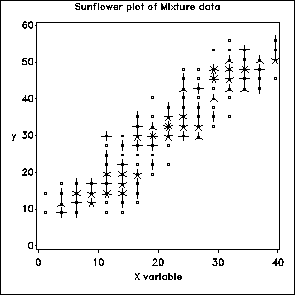sunplot Sunflower plot for X-Y data sunplot

# SAS Macro Programs: sunplot

\$Version: 1.3 (10 Sep 2003)
Michael Friendly
York University

## The sunplot macro (get sunplot.sas) (get sunfont.sas)

### Sunflower plot for X-Y dataset

The sunflower plot displays a bivariate dataset using "sunflower symbols" to show the number of observations in the neighborhood of each XY point.

### Method

The dataset is first "binned" into cells, and the number of observations in each cell is counted. The count in each cell is plotted using the appropriate character from the SUNFONT.

Requires that the program sunfont.sas must first be run to create the sunflower 'sun' font in the libname designated by gfont0.

## Usage

sunplot is a macro program. Values must be supplied for the X and Y variables.

The arguments may be listed within parentheses in any order, separated by commas. For example:

```   %sunplot(data=inputdataset, x=income, y=prestige );
```

### Parameters

Default values are shown after the name of each parameter.
DATA=_LAST_
The name of the input dataset. If not specified, the most recently created dataset is used.
X=X
name of the horizontal variable
Y=Y
name of the vertical variable
NX=20
number of slices of the horizontal var
NY=20
number of slices of the vertical var
COLOR=BLACK
color for plotting symbols
HSYM=1.5
symbol height
VAXIS=
optional name of axis statement for vertical axis
HAXIS=
optional name of axis statement for horizontal axis
PLOTOPT=NOLEGEND FRAME
NAME=SUN
nsme of graphics catalog entry

### Dependencies

You also need to obtain and run sunfont.sas (once) to create the sunflower font. This font is stored in the library (folder) referenced by the LIBNAME GFONT0.

### Example

```%include macros(sunplot);        *-- or include in an autocall library;
data suntest;
label x='X variable';
drop i;
do gp=1 to 3;
mx = 10*gp;
my = mx;
do i = 1 to 100;
x = round((mx + 5 * normal(1242421)), .5);
y = round((my + 3 * normal(2424243) + .5*x), .5);
output;
end;
end;
run;

title1 h=1.5  f=duplex 'Sunflower plot of Mixture data';
axis1 order = 0 to 60 by 10  label=(h=1.5) value=(h=1.5)
minor = none;
axis2 order = 0 to 40 by 10  label=(h=1.5) value=(h=1.5)
minor = none;

%sunplot(data=suntest, x=X, y=Y, nx=20, ny=20, vaxis=axis1, haxis=axis2);

```